Next:Entropy of Degree andUp:Properties of Unified Conditional

## Unified (r,s)-Mutual Information

In this section, we present six different ways to define the unified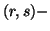mutual information.

Let us consider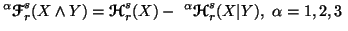and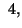(6.6)
and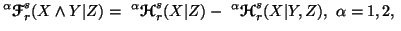and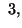(6.7)
where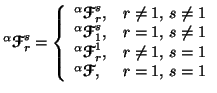(6.8)
and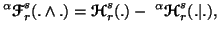etc.We call the measures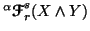(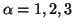and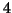) the unifiedmutual information among the random variables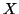and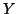. The measures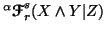(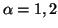and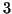) we call the unifiedmutual information among the random variablesandgiven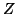.

Property 6.14. We have

(i)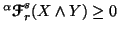(resp.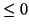) (and), with equality iffandare independent.
(ii)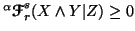(resp.) (and), with equality iffandare independent given.
The above property 6.12 holds under the following conditions:
(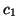) For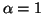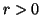with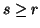or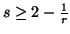(resp.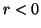with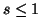or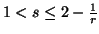);
(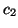) For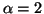and 3,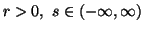(resp.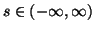);
(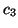) For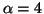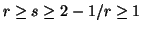(resp.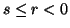) (only for part(i)).
Note 6.4. Similar to Shannon's entropy (property 1.51) we left for the readers to check the validity of the following inequality: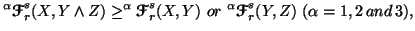i.e., to find the conditions on the parameters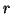and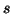for the validity of the above expression.

Property 6.15. We have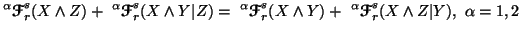and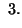(6.9)
Note 6.5. The relation (6.9) is famous as "additive property". It also hold when, but at moment, we are not aware of the conditons onandfor which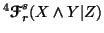is nonnegative, but the particular case when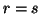is discussed in section 6.4

For Simplicity, let us write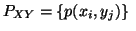and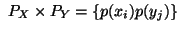forall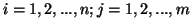.

We have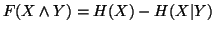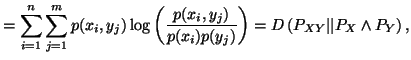where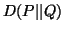is as given in (2.1)

We can write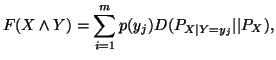where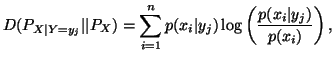for all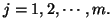Thus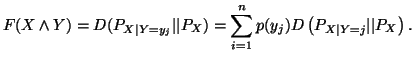The two more ways to define the unifiedmutual information are given by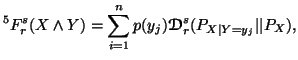(6.10)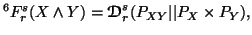(6.11)
where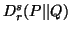is as given by (4.1).

Property 6.16. For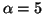and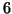, we have

(i)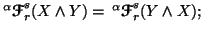(ii)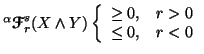with equality iffandare independent.
(iii)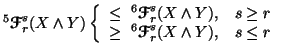Note 6.6. If we consider the fifth and sixth way of unifiedconditional entropies in the following way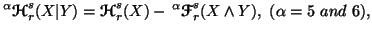(6.12)
then, we don't know for what values ofandthe measures given in (6.12) turn out to be nonnegative.

Note 6.7. Some of the above generalized mutual information measures can be connected to unifieddivergence measures given in Chapter 5. These connections are as follows:

In (5.12), take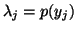and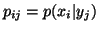, we get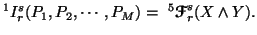Similarly, we can write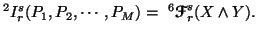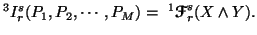In the notes 6.4 and 6.5, we have seen that there are difficulties in getting certain values ofandfor which the measuresor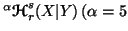and) become nonnegative. But, in the particular case, when, the above difficulties are overcomed. The particular case foris discussed in the following subsection.

21-06-2001
Inder Jeet Taneja
Departamento de Matemática - UFSC
88.040-900 Florianópolis, SC - Brazil